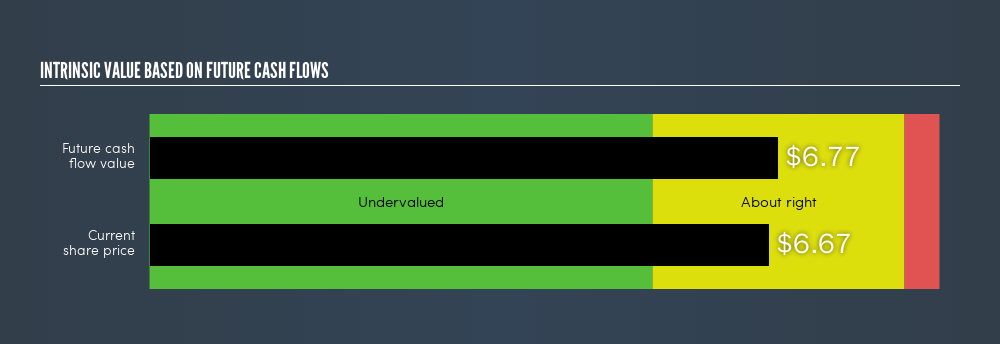### A Look At The Intrinsic Value Of China Distance Education Holdings Limited

04/18/2019Today we will run through one way of estimating the intrinsic value of China Distance Education Holdings Limited (NYSE:DL) by taking the expected future cash flows and discounting them to today’s value. I will be using the Discounted Cash Flow (DCF) model. It may sound complicated, but actually it is quite simple!

What’s the value?

We are going to use a two-stage DCF model, which, as the name states, takes into account two stages of growth. The first stage is generally a higher growth period which levels off heading towards the terminal value, captured in the second ‘steady growth’ period. In the first stage we need to estimate the cash flows to the business over the next ten years. Where possible we use analyst estimates, but when these aren’t available we extrapolate the previous free cash flow (FCF) from the last estimate or reported value. We assume companies with shrinking free cash flow are will slow their rate of shrinkage, and that companies with growing free cash flow will see their growth rate slow, over this period. We do this to reflect that growth tends to slow more in the early years than it does in later years.

Generally we assume that a dollar today is more valuable than a dollar in the future, so we need to discount the sum of these future cash flows to arrive at a present value estimate:

#### 10-year free cash flow (FCF) forecast

 2019 2020 2021 2022 2023 2024 2025 2026 2027 2028 Levered FCF (\$, Millions) US\$56.0 US\$34.1 US\$22.7 US\$17.6 US\$15.0 US\$13.6 US\$12.8 US\$12.3 US\$12.2 US\$12.1 Growth Rate Estimate Source Analyst x1 Analyst x1 Est @ -33.24% Est @ -22.45% Est @ -14.89% Est @ -9.61% Est @ -5.91% Est @ -3.31% Est @ -1.5% Est @ -0.23% Present Value (\$, Millions) Discounted @ 9.36% US\$51.2 US\$28.5 US\$17.4 US\$12.3 US\$9.6 US\$7.9 US\$6.8 US\$6.0 US\$5.4 US\$5.0

Present Value of 10-year Cash Flow (PVCF)= \$150.18m

“Est” = FCF growth rate estimated by Simply Wall St

The second stage is also known as Terminal Value, this is the business’s cash flow after the first stage. For a number of reasons a very conservative growth rate is used that cannot exceed that of a country’s GDP growth. In this case we have used the 10-year government bond rate (2.7%) to estimate future growth. In the same way as with the 10-year ‘growth’ period, we discount future cash flows to today’s value, using a cost of equity of 9.4%.

Terminal Value (TV) = FCF2029 × (1 + g) ÷ (r – g) = US\$12m × (1 + 2.7%) ÷ (9.4% – 2.7%) = US\$188m

Present Value of Terminal Value (PVTV) = TV / (1 + r)10 = \$US\$188m ÷ ( 1 + 9.4%)10 = \$76.85m

The total value is the sum of cash flows for the next ten years plus the discounted terminal value, which results in the Total Equity Value, which in this case is \$227.04m. In the final step we divide the equity value by the number of shares outstanding. This results in an intrinsic value estimate of \$6.77. Relative to the current share price of \$6.67, the company appears about fair value at a 1.4% discount to what it is available for right now. DCFs are imprecise instruments though, rather like a telescope – move a few degrees and end up in a different galaxy. Do keep this in mind.NYSE:DL Intrinsic value, April 17th 2019

The assumptions

We would point out that the most important inputs to a discounted cash flow are the discount rate and of course the actual cash flows. Part of investing is coming up with your own evaluation of a company’s future performance, so try the calculation yourself and check your own assumptions. The DCF also does not consider the possible cyclicality of an industry, or a company’s future capital requirements, so it does not give a full picture of a company’s potential performance. Given that we are looking at China Distance Education Holdings as potential shareholders, the cost of equity is used as the discount rate, rather than the cost of capital (or weighted average cost of capital, WACC) which accounts for debt. In this calculation we’ve used 9.4%, which is based on a levered beta of 1.112. Beta is a measure of a stock’s volatility, compared to the market as a whole. We get our beta from the industry average beta of globally comparable companies, with an imposed limit between 0.8 and 2.0, which is a reasonable range for a stable business.

Source